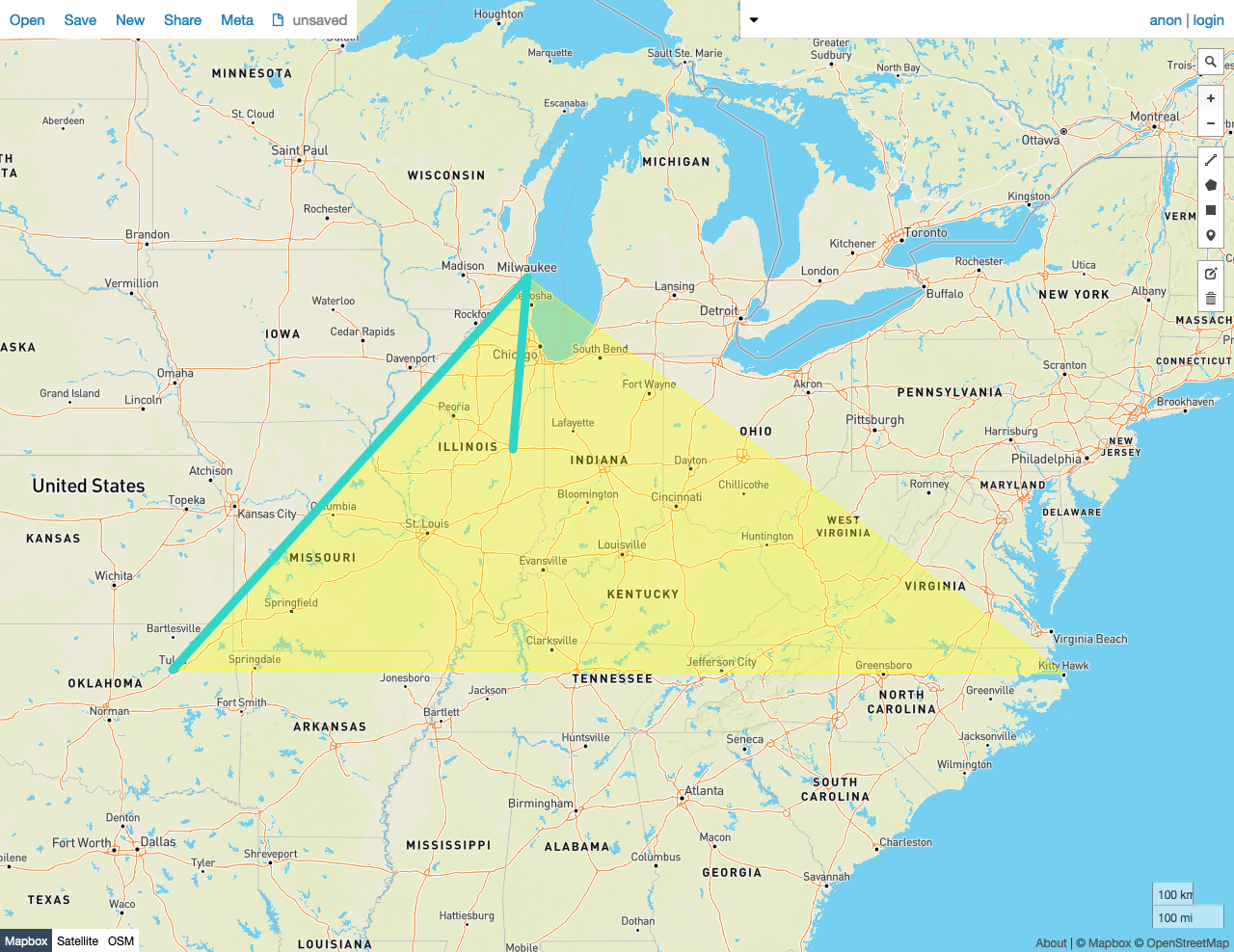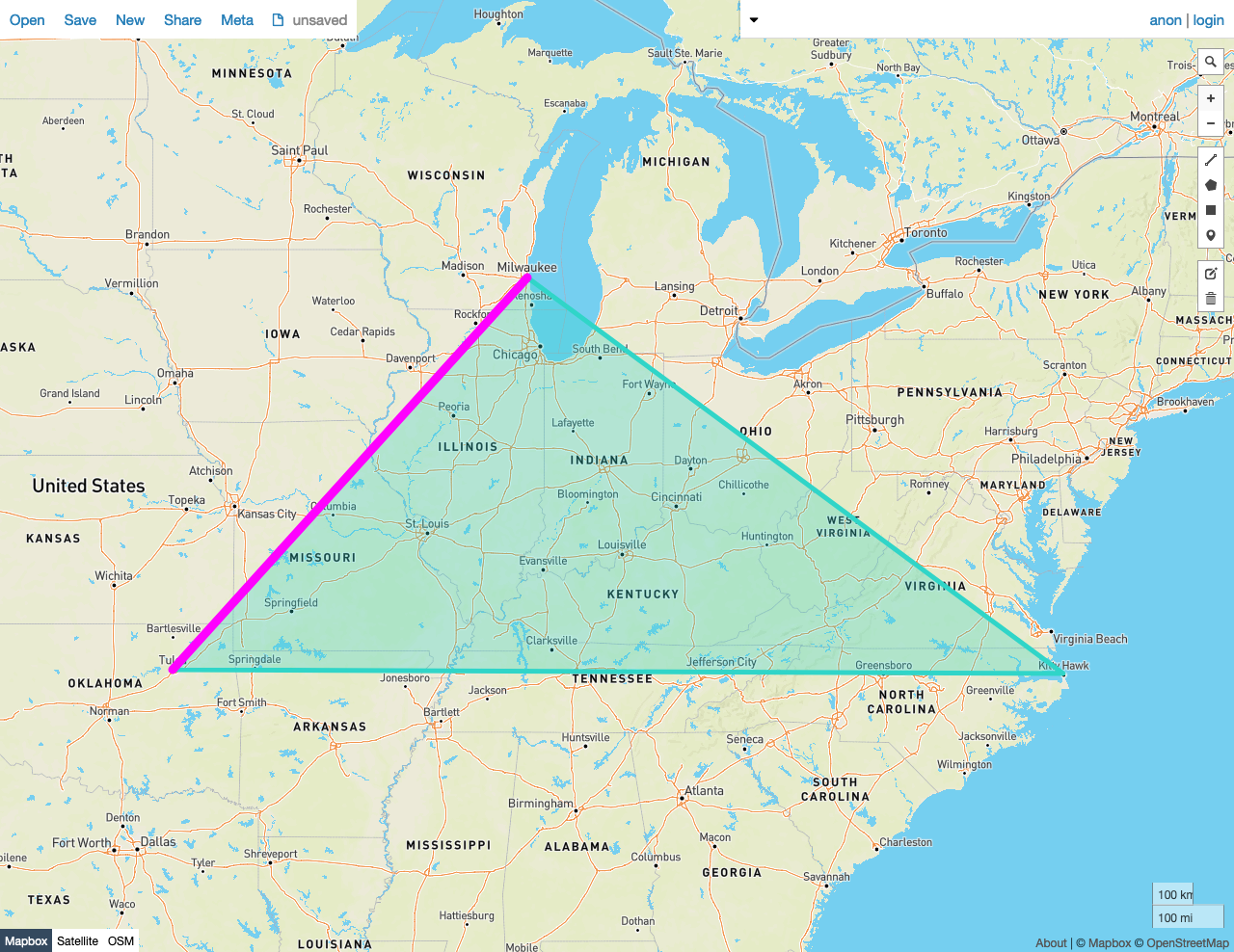Given two shapes A and B, the predicate function `ST_Contains(A, B)` returns `true` if:

• No point in B lies outside of shape A, and
• At least one point in the interior of B lies in the interior of A.

In other words, the exterior of shape A must not include any point in B, and one or more points of B's interior must lie in the interior of A.

This behavior is similar to `ST_Covers`, except that the criteria are more exacting, and therefore some pairs of shapes will be rejected by this function that would be accepted by `ST_Covers`.

`ST_Contains` works on the following data types:

Note:

`ST_Contains` will attempt to use any available spatial index to speed up its operation. Use the prefixed variant `_ST_Contains` if you do not want any spatial indexes to be used.

Note:

This function is the inverse of `ST_Within`.

## Examples

Note:

The screenshots in these examples were generated using geojson.io, but they are designed to showcase the shapes, not the map. Representing `GEOMETRY` data in GeoJSON can lead to unexpected results if using geometries with SRIDs other than 4326 (as shown below).

### True

In this example, `ST_Contains` returns `true` because:

• No point in the LineString B lies outside of the Polygon A, and
• At least one point in the interior of B lies in the interior of A.
``````SELECT ST_Contains(st_geomfromtext('SRID=4326;POLYGON((-87.906471 43.038902, -95.992775 36.153980, -75.704722 36.076944, -87.906471 43.038902))'), st_geomfromtext('SRID=4326;LINESTRING(-88.243385 40.116421, -87.906471 43.038902, -95.992775 36.153980)'));
``````
``````  st_contains
---------------
true

(1 row)
``````### False

In this example, `ST_Contains` returns `false` because:

• At least one point in the interior of LineString B does not lie in the interior of the Polygon A.

Note that A query against these shapes with `ST_Covers` will return `true`.

``````SELECT st_contains(st_geomfromtext('SRID=4326;POLYGON((-87.906471 43.038902, -95.992775 36.153980, -75.704722 36.076944, -87.906471 43.038902))'), st_geomfromtext('SRID=4326;LINESTRING( -87.906471 43.038902, -95.992775 36.153980)'));
``````
``````  st_contains
---------------
false
(1 row)
``````